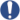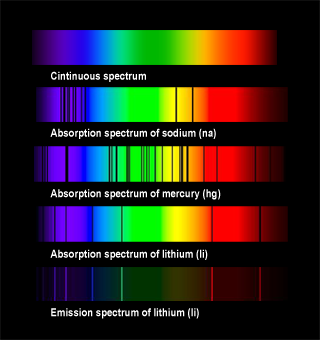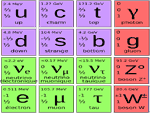# Cosmological constants

## ConstantsAutomatic translation Category: matter and particles
Updated June 01, 2013

Why do we need constant?
To study the universe in its entirety from the infinitely small (with accelerators) to the infinitely large (with telescopes).
The constants are used as tools to test the limits of our scientific theories (theories of Newtonian gravitation, Newtonian Gravitation Quantum, Quantum Mechanics, Quantum Electrodynamics, Special Relativity, Newtonian Mechanics, General Relativity).
In the 17th century Newton wrote the "Principia" (1687), many consider it is the foundation of modern physics because it introduces the concepts of space, time, gravitation and Universe in the sense that the laws are unified. For modern physics to develop, it needs the universal laws to be able to repeat the experience, here and elsewhere, today and tomorrow. These universal laws are exercised within a framework that is the universe. It was at this period that we move from the notion of world to the concept of universe.
The constants thus play a central role in physical theories. Paradoxically constants can vary over very large periods of time, however, the constants are used to structure the domain of validity of the different physical and astrophysical theories.
Jean-Philippe UZAN (IPA) class them into 3 categories:
- The physical constants characteristic of a given system, for example the mass of the electron.
- The constants describe a class of phenomena, such as the gravitational constant.
- The universal constants that appear in many physical laws, such as the speed of light, although the speed of light can be classified in the class of electromagnetic phenomena.
In summary, characterize a constant among these three classes, depending on the knowledge that we have of physics, said Jean-Philippe UZAN.
The three fundamental constants of nature are:
- The speed of light (Electromagnetism, relativity) is a speed limit for the propagation of information, a speed that can not be exceeded.
- The gravitational constant (Gravitation) is a constant of attraction force between the body, the force of attraction between two massive bodies is proportional to the product of their masses and inversely proportional to the square of the distance between their center of mass respective.
- Planck's constant (quantum mechanics) describes the size of quanta. This constant is used in quantum mechanics to link particular the energy of a photon to its frequency. In the standard model and general relativity, we have 20 constants. In addition to these constants, three fundamental units of physics, which are kilogram, meter and second are used. The value of a constant is closely related to the arbitrary value of the meter, the kilogram and the second. That is why we rather define reports, reports of mass or reports of force to avoid miscalculations. With constant, for example, by measuring the distance between the Earth and the Moon, we can test the universality of free fall. We can verify that the Earth and Moon fall in the same way in the gravitational field. With constant, one may wonder if the absorption spectrum of the different elements is the same that there are 10 billion years. Scientists made the measurement and electromagnetic interaction was lower than 0.001%. Only the outer layer of the star is visible, it is the only source of information about the star. Also, to know the constitution of a star we look at the electromagnetic interaction between the light emitted by the star and the elements it pass through. The light emitted by the photosphere is that of an incandescent body, its spectrum is continuous. But in reality, the spectrum observed from the Earth shows stripes.

The continuum spectrum is streaked by numerous absorption lines. A part of light emitted is absorbed by the photosphere. So this absorption is the signature of the chemical elements in the atmosphere of the star.

 Constants Value Speed ​​of light The speed of light in vacuum, c rated for celerity, is a physical constant. The speed of light is the speed of propagation of a wave phenomenon. Its value was set at 299 792 458 m / s in 1983 by the International Bureau of Weights and Measures. c 299 792 458 m/s astronomical unit The astronomical unit is a unit used to measure distances between objects in the solar system. This unit, established in 1958, represents the distance between the Earth and the Sun. Since the 28th General Assembly of the International Astronomical Union (2012), the astronomical unit is exactly 149 597 870 700 m. au 149 597 870 700 m Light year A light year is a unit of distance used in astronomy. A light-year is equal to the distance that light travels in a vacuum in the space of one year (31,557,600 seconds), about 10,000 billion kilometers. a.l. 9 460 895 288 762 850 m Parsec The parsec is a unit of length used in astronomy. Its name comes from the contraction of "parallax-second." The parsec is defined as the distance at which one astronomical unit (AU) subtends an angle of one arc second. A parsec equals 206 270.6904 au or 3.2616 light years or 30 857 656 073 828 900 m. pc 30 857 656 073 828 900 m Gravitational constant The gravitational constant G is a constant of the force of the attraction between bodies, the force of attraction between two massive bodies is proportional to the product of their masses and inversely proportional to the square of the distance between the respective center of mass. G 6,673 84×10-11 m³.kg-1.s-2 Cosmological constant The cosmological constant is the average energy density of the vacuum on cosmological scales. The magnitude of this constant is inconnue.En 1917, Einstein added a parameter to its equations of general relativity (1915), he called the constant cosmological this to make the theory compatible with the vision of a static universe. Since 1929 we know, thanks to Edwin Hubble that the universe is expanding because when the electromagnetic spectrum all distant cosmic objects analysis, oberserve a redshift. Λ free parameter 0 or ≠0 Boltzmann constant The Boltzmann constant (k or kB), the name of Ludwig Boltzmann, is a physical constant that links the energy level of the individual particle, with temperature. This fundamental physical constant is equal to R / N. R is the gas constant. N is Avogadro's number equal to N = 6.022 × 1023 mol -1 ), the number of particles in a mole. The sensation of heat or cold is actually a transfer of energy from one body to another in the form of heat. Entropy derived from k. k 1,3806488×10-23 J.K-1 Hubble constant Hubble constant (H0) is a cosmological constant of proportionality between distance and apparent rate of recession of the galaxies in the observable universe. It is connected to the famous Hubble's Law describing the expansion of the universe. The Hubble constant gives the current expansion rate of the universe. The observations give an approximate value of 73 km / s / Mpc. For example, a galaxy located 1 megaparsec (about 3.26 million light years) moves away due to the expansion of the universe (excluding the effect of the proper motion of the object is negligible at high distance), at a speed of 73 km / s, a 10 galaxy Mpc moves away at a speed of 730 m / s... h0 73 km/s/Mpc Planck constant Planck constant h, describes the size of quanta. This constant is used in quantum mechanics to link in particular the energy of a photon to its frequency. h 6,62606957×10-34 J.s Planck length The Planck length is generally described as the length from which the gravity would begin to exhibit quantum effects, which would require a theory of quantum gravity to be described. Planck length would be the minimum length that is possible to measure significantly. In superstring theory, the Planck length sets the minimum diameter of a string. lp 1,616 24(12)×10-35 m Planck time Planck time is the time it would take a photon in a vacuum to travel a distance equal to the Planck length. Since the Planck length is the smallest measurable length and the speed of light the fastest possible speed, the Planck time is the smallest time measurement with a physical meaning in the context of our theories. The age of the universe is estimated at about 14 billion years, or 4x1017 seconds. Therefore approximately sixty orders of magnitude separate the Planck scale of the cosmic scale. Our current fundamental theories, quantum mechanics and general relativity, are unable to include in a unified scheme quantities so disproportionate. tp 5,19121 71(40)×10-44 s Planck mass Planck mass or the mass of a Planck particle is the hypothetical tiny black hole whose Schwarzschild radius is equal to the Planck length. Unlike all other base units Planck and most Planck units derived the Planck mass has a more or less conceivable human scale. Tradition has it that this is the mass of the egg of the flea. The Planck mass is an idealized mass thought to have a special significance for quantum gravity. mp 1,672 621 71(29)×10-27 kg Planck temperaturePlanck temperature is the highest temperature in the physical theories. At one end of the temperature scale was the lowest temperature possible, the absolute zero (0 K) and on the other the highest possible temperature, which is the Planck equal to 1.416 x 10 32 K. This temperature corresponds to the temperature of the Universe at the time called Planck time is 10 -44 seconds after the bigbang. Tp 1.41679 x 1032 K Fine-structureconstant In physics, the fine structure constant, represented by the Greek letter α is a fundamental constant that governs the electromagnetic force ensuring consistency of atoms and molecules. It was proposed in 1916 by the German physicist Arnold Sommerfeld. In quantum electrodynamics, the fine structure constant serves as the coupling constant, representing the strength of interaction between the electrons and photons. Its value can not be predicted by the theory, but only determined by experimental results. This is actually one of the 29 free parameters of the Standard Model of particle physics. α 7,2973525698(24)×10-3 Proton mass The nuclei of Atoms are composed of protons and neutrons. around these nuclei, revolves a cloud of electrons. These three elements (protons, neutrons and electrons) are practically all matter. While the electron is considered as a particle "no size", the proton, which is made up of quarks, is an "extended" object. mn 1,674 927 28(29)×10-27 kg Electron mass The electron is an elementary particle that has a charge of negative sign. This is one of the components of the atom with neutrons and protons but the electron is rather a kind of electric point weighing, which no one knows or where he is or where it goes. He turns on itself like a spinning top without stopping and it has some form of connivance very discrete (weak interaction) with many other particles. me 9,109 382 6(16)×10-31 kg Muon mass In the standard model of particle physics, the muon is an elementary particle with a negative charge. The muon has the same physical properties as the electron, but with a mass 207 times larger, it is also called heavy electron. mµ 1,883 531 40(33)×10-28 kg Tau mass Tau or tauon is a particle of the lepton family. its properties are close to those of the electron and the muon, but it is more massive and short-lived. With its associated neutrino and t quarks (top) and b (bottom or beauty), it forms the third series (the most massive) of fermions in the standard model. mτ 3,167 77(52)×10-27 kg Boson mass Z0 The Gluon is the mediator of the strong interaction, ie of the nuclear force, the photon is the mediator of the electromagnetic interaction, the weak interaction but still has no mediator. The physicist Peter Higgs imagined one in the 1960s. This hypothetical particle called Boson. Thus the Higgs mechanism fills the entire universe and all space, molasses, a field of bosons. mz° 1,625 56(13)×10-25 kg Boson mass W The Gluon is the mediator of the strong interaction, ie of the nuclear force, the photon is the mediator of the electromagnetic interaction, the weak interaction but still has no mediator. The physicist Peter Higgs imagined one in the 1960s. This hypothetical particle called Boson. Thus the Higgs mechanism fills the entire universe and all space, molasses, a field of bosons. mw 1,4334(18)×10-25 kg

nota: Meter (symbol m) is the length of the path traveled in a vacuum by light during 1/299 792 458 second.

nota: Kilogram (symbol kg) is the base unit of mass in the International System of Units (SI). It is defined as being equal to the mass of the international prototype of the kilogram. This is the only unit that is defined by a physical object and not a fundamental physical property.

nota: Second (symbol s) is the duration of 9,192,631,770 periods of the radiation corresponding to the transition between the hyperfine levels of the fundamental state of the cesium 133 atom. It is a multiple of the period of the wave emitted by a cesium-133 atom when its electrons change energy level.

nota: Scientists of quantum mechanics: Ludwig Boltzmann, Max Planck, Albert Einstein, Niels Bohr, Louis de Broglie, Werner Heisenberg, Erwin Schrödinger and Paul Dirac.
Scientists of general relativity: Alex Grossmann, Willem de Sitter, Albert Einstein, Arthur Stanley Eddington, Karl Schwarzschild, Alexander Friedmann, Georges Lemaitre.### Image: Absorption lines of the chemical elements. When a chemical element is crossed by white light, the color spectrum that reaches us, shows us black lines. These lines are the signature of the chemical elements traversed by the light. So we can know the chemical composition of a star because the white light from its photosphere through the ions present in the atmosphere. For a same elements, the absorption lines correspond to the emission lines (see the two spectra at the bottom of the image). As a chemical element absorbs radiation that is capable of transmitting, the absorption and emission lines have the same wavelength. The black lines in the absorption spectrum of lithium correspond with the colored lines of the emission spectrum.Noncommercial, educational use only.

nota: The three units of measurement useful in astronomy to express the distances:
-
light year (a.l.) A light year is a unit of distance used in astronomy. A light-year is equal to the distance that light travels in a vacuum in the space of one year (31,557,600 seconds), about 10,000 billion kilometers. is the 63242.17881 au, is exactly equal to 9 460 895 288 762 850 m.
-
parsec (pc The parsec is the distance at which one astronomical unit subtends an angle of one arcsecond.) is equal to 206 270,6904 UA or 3.2616 years-light or 30 857 656 073 828 900 m.
-
astronomical unit (au (symbol: ua ou au) Créée en 1958, c’est l'unité de distance utilisée pour mesurer les distances des objets du système solaire, cette distance est égale à la distance de la Terre au Soleil. La valeur de l'unité astronomique représente exactement 149 597 870 700 m, lors de son assemblée générale tenue à Pékin, du 20 au 31 août 2012, l'Union astronomique internationale (UAI) a adopté une nouvelle définition de l'unité astronomique, unité de longueur utilisée par les astronomes du monde entier pour exprimer les dimensions du Système solaire et de l’Univers. On retiendra environ 150 millions de kilomètres. Une année-lumière vaut approximativement 63 242 ua. Mercure: 0,38 ua, Vénus: 0,72 ua, Terre: 1,00 ua, Mars: 1,52 ua, Ceinture d’astéroïdes: 2 à 3,5 ua, Jupiter: 5,21 ua, Saturne: 9,54 ua, Uranus: 19,18 ua, Neptune: 30,11 ua, Ceinture de Kuiper: 30 à 55 ua, Nuage d’Oort: 50 000 ua.) is from August 30, 2012, exactly 149 597 870 700 meters.

Table of equivalences on units of distances:

 pc al au km pc 1 3,26 206265 3,09x1013 al 0,307 1 63242 9,46x1012 au 4,85x10-6 1,58x10-5 1 1,50x108 km 3,24x10-14 1,06x10-13 6,68x10-9 1

## Videos on the cosmological constant

Conferences: Institute of Astrophysics of Paris (IAP)
Release Date: January 1, 2002
Autor(s): UZAN Jean-Philippe
Conferences: Institute of Astrophysics of Paris (IAP)
Release Date: April 9, 2013
Autor(s): COLLIN-ZAHN Suzy, VILAIN Christiane
Conferences: Institute of Astrophysics of Paris (IAP)
Release Date: April 5, 2011
Autor(s): Nathalie Palanque-Delabrouille

1997 © Astronoo.com − Astronomy, Astrophysics, Evolution and Earth science.Closer to the matterimage of the atom...The first secondof our history...Special relativity,space and time...Principle of atomicabsorption and emission...12 particles of matter...